# ORF523: Noisy oracles

Today we start the third (and last) part of our class titled: ‘Optimization and Randomness’. An important setting that we will explore is the one of optimization with noisy oracles:

• Noisy-order oracle: given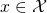it outputssuch that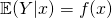.
• Noisy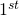-order oracle: givenit outputs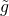such that.

Somewhat surprisingly several of the first-order algorithms we studied so far are robust to noise and attain the same performance if one feeds them with the answers of a noisyorder oracle. This was first observed by Robbins and Monro in the early Fifties, and the resulting set of techniques is often referred to as stochastic approximation methods. For instance let us consider the Stochastic Mirror Descent:The analysis of this algorithm follows the exact same lines than the standard version. The key observation is that: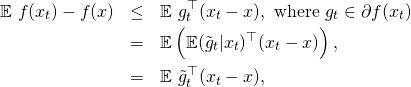and now one can repeat the standard proof sincewas used in the algorithm. This yields the following result.

Theorem. Letbe a mirror map-strongly convex onwith respect to, and let. Assume thatis convex and that the noisy oracle is such that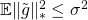. Then Stochastic Mirror Descent withsatisfies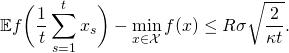Of course the exact same reasoning applies to the Mirror Descent for Saddle Points. One can also get the ratefor strongly convex functions using the idea described in this lecture. High-probability results can be obtained using Markov’s inequality, and stronger concentration results can be proved under a subgaussian assumption on the tails of the estimates, see Proposition 2.2. here.

Let us now see some application of this noisy framework.

Statistical Learning

We are interested in the solving the following problem:whereis a known loss function such thatis a convex function, andis a random variable whose distribution is unknown. While the distribution ofis unknown, we assume that we have access to a sequence of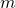i.i.d. examples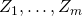. A typical example within this framework is the case where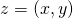represents a pair of (input, output) and the function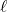is one of the loss function we saw in this lecture, for example the logistic losswith,.

Letbe a subgradient ofat, and let. We assume that. Note that we cannot perform a gradient descent onas we would need to know the distribution ofto compute a subgradient of. On the other hand one has access to unbiased estimates of these subgradients with the i.i.d. examples. Thus one can use the Stochastic Mirror Descent, where at each iteration a new example is used to compute the noisy subgradient, precisely we take. In particular withexamples one can only run the algorithm forsteps, and one gets to a precision of order. If in additionis strongly convex then one can in fact obtain a precision of orderwith the step sizes/averaging described in this lecture.

Acceleration by randomization

In the previous example there was no alternative to the use of noisy subgradients as the distribution ofwas unknown. However in some cases, even if the distribution ofis known, it might be beneficial to artificially sample examples from the distribution in order to avoid computing difficult integrals. Consider for example the problem of minimizing an average of convex functions: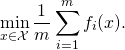We have seen several important examples of this form wheremight be very large. Note that computing a subgradient oftakestime where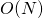is the time to compute the subgradient of a single function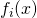. Thus a standard gradient descent algorithm will take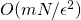time to reach an-optimal point. On the other hand one can simply sample (with replacement) an indexat every iteration (which takes time), and perform a step of gradient descent using only the subgradient of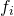. This method will reach an-optimal point (in expectation) in time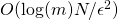, which is much cheaper than the requirement for GD!

Note that ifis also strongly convex, then GD needs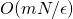time to reach an-optimal point, and SGD needs onlytime (with appropriate step sizes/averaging as discussed above). The situation becomes more favorable to GD if in additionis smooth (recall that for strongly convex and smooth functions one has an exponential rate of convergence for GD), and we will come back to this in the next lecture.

Let us consider another example which we studied at the end of the previous lecture: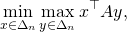where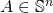(the symmetry assumption is just for sake of notation). It is clear that the rate of convergence of MD-SP for this problem is of order. Furthermore each step requires to compute a subgradient inand, which are of the form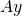and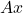. Thus the time complexity of one step is of order(which is the time complexity of a matrix-vector multiplication). Overall to reach an-optimal points one needselementary operations. Now the key observation is that the matrix-vector multiplication can easily be randomized, especially since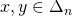. Let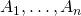be the column vectors of, then, and ifis a random variable such thatone has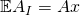. Using this randomization in a Stochastic MD-SP one obtains the exact same rate of convergence (in expectation) but now each step has time complexity of order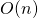, resulting in an overall complexity to reach an-optimal point (in expectation) of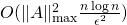. This result is especially amazing since we obtain a sublinear time algorithm (the complexity is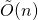while the ‘size’ of the problem is). One can propose an even better algorithm based on Mirror Prox with a complexity ofand we refer the reader to this book chapter by Juditsky and Nemirovski for more details.

This entry was posted in Optimization. Bookmark the permalink.